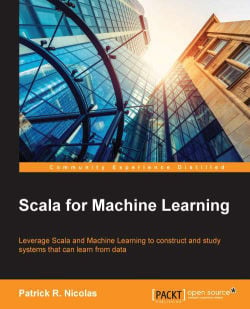# Scala for Machine Learning4.3 (4 reviews total)
By Patrick R. Nicolas
What do you get with a Packt Subscription?

• Constantly updated with 100+ new titles each month
• Breadth and depth in over 1,000+ technologies

The discovery of information through data clustering and classification is becoming a key differentiator for competitive organizations. Machine learning applications are everywhere, from self-driving cars, engineering designs, biometrics, and trading strategies, to detection of genetic anomalies.

The book begins with an introduction to the functional capabilities of the Scala programming language that are critical to the creation of machine learning algorithms such as dependency injection and implicits.

Next, you'll learn about data preprocessing and filtering techniques. Following this, you'll move on to clustering and dimension reduction, Naïve Bayes, regression models, sequential data, regularization and kernelization, support vector machines, neural networks, generic algorithms, and re-enforcement learning. A review of the Akka framework and Apache Spark clusters concludes the tutorial.

Publication date:
December 2015
Publisher
Packt
Pages
520
ISBN
9781783558742

## Chapter 1. Getting Started

It is critical for any computer scientist to understand the different classes of machine learning algorithms and be able to select the ones that are relevant to the domain of their expertise and dataset. However, the application of these algorithms represents a small fraction of the overall effort needed to extract an accurate and performing model from input data. A common data mining workflow consists of the following sequential steps:

1. Defining the problem to solve.

3. Preprocessing, analyzing, and filtering the input data.

4. Discovering patterns, affinities, clusters, and classes, if needed.

5. Selecting the model features and appropriate machine learning algorithm(s).

6. Refining and validating the model.

7. Improving the computational performance of the implementation.

In this book, each stage of the process is critical to build the right model.

### Tip

It is impossible to describe the key machine learning algorithms and their implementations in detail in a single book. The sheer quantity of information and Scala code would overwhelm even the most dedicated readers. Each chapter focuses on the mathematics and code that are absolutely essential to the understanding of the topic. Developers are encouraged to browse through the following:

• The Scala coding convention and standard used in the book in the Appendix A, Basic Concepts

• API Scala docs

• A fully documented source code that is available online

This first chapter introduces you to the taxonomy of machine learning algorithms, the tools and frameworks used in the book, and a simple application of logistic regression to get your feet wet.

## Mathematical notation for the curious

Each chapter contains a small section dedicated to the formulation of the algorithms for those interested in the mathematical concepts behind the science and art of machine learning. These sections are optional and defined within a tip box. For example, the mathematical expression of the mean and the variance of a variable X mentioned in a tip box will be as follows:

### Tip

Convention and notation

This book uses zero-based indexing of datasets in the mathematical formulas.

M1: A set of N observations is denoted as {xi} = x0, x1, … , xN-1, and the arithmetic mean value for the random value with xi as values is defined as: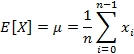## Why machine learning?

The explosion in the number of digital devices generates an ever-increasing amount of data. The best analogy I can find to describe the need, desire, and urgency to extract knowledge from large datasets is the process of extracting a precious metal from a mine, and in some cases, extracting blood from a stone.

Knowledge is quite often defined as a model that can be constantly updated or tweaked as new data comes into play. Models are obviously domain-specific ranging from credit risk assessment, face recognition, maximization of quality of service, classification of pathological symptoms of disease, optimization of computer networks, and security intrusion detection, to customers' online behavior and purchase history.

Machine learning problems are categorized as classification, prediction, optimization, and regression.

### Classification

The purpose of classification is to extract knowledge from historical data. For instance, a classifier can be built to identify a disease from a set of symptoms. The scientist collects information regarding the body temperature (continuous variable), congestion (discrete variables HIGH, MEDIUM, and LOW), and the actual diagnostic (flu). This dataset is used to create a model such as IF temperature > 102 AND congestion = HIGH THEN patient has the flu (probability 0.72), which doctors can use in their diagnostic.

### Prediction

Once the model is trained using historical observations and validated against historical observations, it can be used to predict some outcome. A doctor collects symptoms from a patient, such as body temperature and nasal congestion, and anticipates the state of his/her health.

### Optimization

Some global optimization problems are intractable using traditional linear and non-linear optimization methods. Machine learning techniques improve the chances that the optimization method converges toward a solution (intelligent search). You can imagine that fighting the spread of a new virus requires optimizing a process that may evolve over time as more symptoms and cases are uncovered.

### Regression

Regression is a classification technique that is particularly suitable for a continuous model. Linear (least squares), polynomial, and logistic regressions are among the most commonly used techniques to fit a parametric model, or function, y= f (x), x={xi}, to a dataset. Regression is sometimes regarded as a specialized case of classification for which the output variables are continuous instead of categorical.

## Why Scala?

Like most functional languages, Scala provides developers and scientists with a toolbox to implement iterative computations that can be easily woven into a coherent dataflow. To some extent, Scala can be regarded as an extension of the popular MapReduce model for distributed computation of large amounts of data. Among the capabilities of the language, the following features are deemed essential in machine learning and statistical analysis.

### Abstraction

Functors and monads are important concepts in functional programming. Monads are derived from the category and group theory that allow developers to create a high-level abstraction as illustrated in Scalaz, Twitter's Algebird, or Google's Breeze Scala libraries. More information about these libraries can be found at the following links:

In mathematics, a category M is a structure that is defined by:

• Objects of some type: {x ϵ X, y ϵ Y, z ϵ Z, …}

• Morphisms or maps applied to these objects: x ϵ X, y ϵ Y, f: x -› y

• Composition of morphisms: f: x -› y, g: y -› z => g o f: x -› z

Covariant, contravariant functors, and bifunctors are well-understood concepts in algebraic topology that are related to manifold and vector bundles. They are commonly used in differential geometry and generation of non-linear models from data.

#### Higher-kind projection

Scientists define observations as sets or vectors of features. Classification problems rely on the estimation of the similarity between vectors of observations. One technique consists of comparing two vectors by computing the normalized inner product. A co-vector is defined as a linear map α of a vector to the inner product (field).

### Tip

Inner product

M1: The definition of a <.> inner product and a α co-vector is as follows: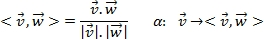Let's define a vector as a constructor from any `_ => Vector[_]` field (or `Function1[_, Vector]`). A co-vector is then defined as the mapping function of a vector to its `Vector[_] => _` field (or `Function1[Vector, _]`).

Let's define a two-dimensional (two types or fields) higher kind structure, `Hom`, that can be defined as either a vector or co-vector by fixing one of the two types:

```type Hom[T] = {
type Right[X] = Function1[X,T] // Co-vector
type Left[X] = Function1[T,X]   // Vector
}```

### Note

Tensors and manifolds

Vectors and co-vectors are classes of tensor (contravariant and covariant). Tensors (fields) are used in manifold learning of nonlinear models and in the generation of kernel functions. Manifolds are briefly introduced in the Manifolds section under Dimension reduction in Chapter 4, Unsupervised Learning. The topic of tensor fields and manifold learning is beyond the scope of this book.

The projections of the higher kind, `Hom`, to the `Right` or `Left` single parameter types are known as functors, which are as follows:

• A covariant functor for the `right` projection

• A contravariant functor for the `left` projection.

#### Covariant functors for vectors

A covariant functor of a variable is a map F: C => C such that:

• If f: x -› y is a morphism on C, then F(x) -› F(y) is also a morphism on C

• If id: x -› x is the identity morphism on C, then F(id) is also an identity morphism on C

• If g: y -› z is also a morphism on C, then F(g o f) = F(g) o F(f)

The definition of the `F[U => V] := F[U] => F[V]`covariant functor in Scala is as follows:

```trait Functor[M[_]] {
def map[U,V](m: M[U])(f: U =>V): M[V]
}```

For example, let's consider an observation defined as a `n` dimension vector of a `T` type, `Obs[T]`. The constructor for the observation can be represented as `Function1[T,Obs]`. Its `ObsFunctor` functor is implemented as follows:

```trait ObsFunctor[T] extends Functor[(Hom[T])#Left] { self =>
override def map[U,V](vu: Function1[T,U])(f: U =>V):
Function1[T,V] = f.compose(vu)
}```

The functor is qualified as a covariant functor because the morphism is applied to the return type of the element of `Obs` as `Function1[T, Obs]`. The `Hom` projection of the two parameters types to a vector is implemented as `(Hom[T])#Left`.

#### Contravariant functors for co-vectors

A contravariant functor of one variable is a map F: C => C such that:

• If f: x -› y is a morphism on C, then F(y) -> F(x) is also a morphism on C

• If id: x -› x is the identity morphism on C, then F(id) is also an identity morphism on C

• If g: y -› z is also a morphism on C, then F(g o f) = F(f) o F(g)

The definition of the `F[U => V] := F[V] => F[U]` contravariant functor in Scala is as follows:

```trait CoFunctor[M[_]] {
def map[U,V](m: M[U])(f: V =>U): M[V]
}```

Note that the input and output types in the `f` morphism are reversed from the definition of a covariant functor. The constructor for the co-vector can be represented as `Function1[Obs,T]`. Its `CoObsFunctor` functor is implemented as follows:

```trait CoObsFunctor[T] extends CoFunctor[(Hom[T])#Right] {
self =>
override def map[U,V](vu: Function1[U,T])(f: V =>U):
Function1[V,T] = f.andThen(vu)
}```

Monads are structures in algebraic topology that are related to the category theory. Monads extend the concept of a functor to allow a composition known as the monadic composition of morphisms on a single type. They enable the chaining or weaving of computation into a sequence of steps or pipeline. The collections bundled with the Scala standard library (`List`, `Map`, and so on) are constructed as monads [1:1].

Monads provide the ability for those collections to perform the following functions:

• Create the collection

• Transform the elements of the collection

• Flatten nested collections

An example is as follows:

```trait Monad[M[_]] {
def unit[T](a: T): M[T]
def map[U,V](m: M[U])(f U =>V): M[V]
def flatMap[U,V](m: M[U])(f: U =>M[V]): M[V]
}```

Monads are therefore critical in machine learning as they enable you to compose multiple data transformation functions into a sequence or workflow. This property is applicable to any type of complex scientific computation [1:2].

### Note

The monadic composition of kernel functions

Monads are used in the composition of kernel functions in the Kernel monadic composition section under Kernel functions section in Chapter 8, Kernel Models and Support Vector Machines.

### Scalability

As seen previously, functors and monads enable parallelization and chaining of data processing functions by leveraging the Scala higher-order methods. In terms of implementation, actors are one of the core elements that make Scala scalable. Actors provide Scala developers with a high level of abstraction to build scalable, distributed, and concurrent applications. Actors hide the nitty-gritty implementation details of concurrency and the management of the underlying threads pool. Actors communicate through asynchronous immutable messages. A distributed computing Scala framework such as Akka or Apache Spark extends the capabilities of the Scala standard library to support computation on very large datasets. Akka and Apache Spark are described in detail in the last chapter of this book [1:3].

In a nutshell, a workflow is implemented as a sequence of activities or computational tasks. These tasks consist of high-order Scala methods such as `flatMap`, `map`, `fold`, `reduce`, `collect`, `join`, or `filter` that are applied to a large collection of observations. Scala provides developers with the tools to partition datasets and execute the tasks through a cluster of actors. Scala also supports message dispatching and routing between local and remote actors. A developer can decide to deploy a workflow either locally or across multiple CPU cores and servers with very few code alterations.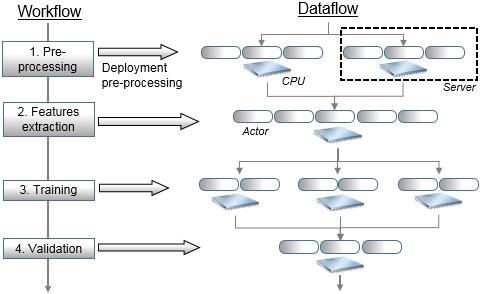Deployment of a workflow for model training as a distributed computation

In the preceding diagram, a controller, that is, the master node, manages the sequence of tasks 1 to 4 similar to a scheduler. These tasks are actually executed over multiple worker nodes, which are implemented by actors. The master node or actor exchanges messages with the workers to manage the state of the execution of the workflow as well as its reliability, as illustrated in the Scalability with Actors section in Chapter 12, Scalable Frameworks. High availability of these tasks is implemented through a hierarchy of supervising actors.

### Configurability

Scala supports dependency injection using a combination of abstract variables, self-referenced composition, and stackable traits. One of the most commonly used dependency injection patterns, the cake pattern, is described in the Composing mixins to build a workflow section in Chapter 2, Hello World!

### Maintainability

Scala embeds Domain Specific Languages (DSL) natively. DSLs are syntactic layers built on top of Scala native libraries. DSLs allow software developers to abstract computation in terms that are easily understood by scientists. The most notorious application of DSLs is the definition of the emulation of the syntax used in the MATLAB program, which data scientists are familiar with.

### Computation on demand

Lazy methods and values allow developers to execute functions and allocate computing resources on demand. The Spark framework relies on lazy variables and methods to chain Resilient Distributed Datasets (RDD).

## Model categorization

A model can be predictive, descriptive, or adaptive.

Predictive models discover patterns in historical data and extract fundamental trends and relationships between factors (or features). They are used to predict and classify future events or observations. Predictive analytics is used in a variety of fields, including marketing, insurance, and pharmaceuticals. Predictive models are created through supervised learning using a preselected training set.

Descriptive models attempt to find unusual patterns or affinities in data by grouping observations into clusters with similar properties. These models define the first and important step in knowledge discovery. They are generated through unsupervised learning.

A third category of models, known as adaptive modeling, is created through reinforcement learning. Reinforcement learning consists of one or several decision-making agents that recommend and possibly execute actions in the attempt of solving a problem, optimizing an objective function, or resolving constraints.

## Taxonomy of machine learning algorithms

The purpose of machine learning is to teach computers to execute tasks without human intervention. An increasing number of applications such as genomics, social networking, advertising, or risk analysis generate a very large amount of data that can be analyzed or mined to extract knowledge or insight into a process, customer, or organization. Ultimately, machine learning algorithms consist of identifying and validating models to optimize a performance criterion using historical, present, and future data [1:4].

Data mining is the process of extracting or identifying patterns in a dataset.

### Unsupervised learning

The goal of unsupervised learning is to discover patterns of regularities and irregularities in a set of observations. The process is known as density estimation in statistics is broken down into two categories: discovery of data clusters and discovery of latent factors. The methodology consists of processing input data to understand patterns similar to the natural learning process in infants or animals. Unsupervised learning does not require labeled data (or expected values), and therefore, it is easy to implement and execute because no expertise is needed to validate an output. However, it is possible to label the output of a clustering algorithm and use it for future classification.

#### Clustering

The purpose of data clustering is to partition a collection of data into a number of clusters or data segments. Practically, a clustering algorithm is used to organize observations into clusters by minimizing the distance between observations within a cluster and maximizing the distance between observations across clusters. A clustering algorithm consists of the following steps:

1. Creating a model by making an assumption on the input data.

2. Selecting the objective function or goal of the clustering.

3. Evaluating one or more algorithms to optimize the objective function.

Data clustering is also known as data segmentation or data partitioning.

#### Dimension reduction

Dimension reduction techniques aim at finding the smallest but most relevant set of features needed to build a reliable model. There are many reasons for reducing the number of features or parameters in a model, from avoiding overfitting to reducing computation costs.

There are many ways to classify the different techniques used to extract knowledge from data using unsupervised learning. The following taxonomy breaks down these techniques according to their purpose, although the list is far from being exhaustive, as shown in the following diagram: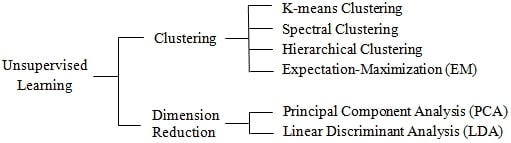Taxonomy of unsupervised learning algorithms

### Supervised learning

The best analogy for supervised learning is function approximation or curve fitting. In its simplest form, supervised learning attempts to find a relation or function f: x → y using a training set {x, y}. Supervised learning is far more accurate than any other learning strategy as long as the input (labeled data) is available and reliable. The downside is that a domain expert may be required to label (or tag) data as a training set.

Supervised machine learning algorithms can be broken into two categories:

• Generative models

• Discriminative models

#### Generative models

In order to simplify the description of a statistics formula, we adopt the following simplification: the probability of an X event is the same as the probability of the discrete X random variable to have a value x: p(X) = p(X=x).

The notation for the joint probability is p(X,Y) = p(X=x,Y=y).

The notation for the conditional probability is p(X|Y) = p(X=x|Y=y).

Generative models attempt to fit a joint probability distribution, p(X,Y), of two X and Y events (or random variables), representing two sets of observed and hidden x and y variables. Discriminative models compute the conditional probability, p(Y|X), of an event or random variable Y of hidden variables y, given an event or random variable X of observed variables x. Generative models are commonly introduced through the Bayes' rule. The conditional probability of a Y event, given an X event, is computed as the product of the conditional probability of the X event, given the Y event, and the probability of the X event normalized by the probability of the Y event [1:5].

### Note

Bayes' rule

Joint probability for independent random variables, X=x and Y=y, is given by: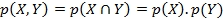Conditional probability of a random variable, Y = y, given X = x, is given by: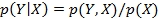Bayes' formula is given by: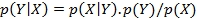The Bayes' rule is the foundation of the Naïve Bayes classifier, as described in the Introducing the multinomial Naïve Bayes section in Chapter 5, Naïve Bayes Classifiers.

#### Discriminative models

Contrary to generative models, discriminative models compute the conditional probability p(Y|X) directly, using the same algorithm for training and classification.

Generative and discriminative models have their respective advantages and disadvantages. Novice data scientists learn to match the appropriate algorithm to each problem through experimentation. Here is a brief guideline describing which type of models make sense according to the objective or criteria of the project:

Objective

Generative models

Discriminative models

Accuracy

Highly dependent on the training set.

This depends on the training set and algorithm configuration (that is, kernel functions)

Modeling requirements

There is a need to model both observed and hidden variables, which requires a significant amount of training.

The quality of the training set does not have to be as rigorous as for generative models.

Computation cost

This is usually low. For example, any graphical method derived from the Bayes' rule has low overhead.

Most algorithms rely on optimization of a convex function with significant performance overhead.

Constraints

These models assume some degree of independence among the model features.

Most discriminative algorithms accommodate dependencies between features.

We can further refine the taxonomy of supervised learning algorithms by segregating arbitrarily between sequential and random variables for generative models and breaking down discriminative methods as applied to continuous processes (regression) and discrete processes (classification):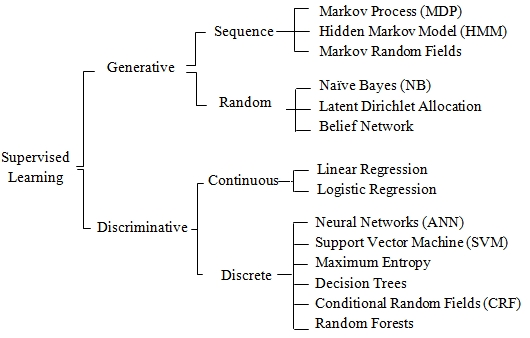Taxonomy of supervised learning algorithms

### Semi-supervised learning

Semi-supervised learning is used to build models from a dataset with incomplete labels. Manifold learning and information geometry algorithms are commonly applied to large datasets that are partially labeled. The description of semi-supervised learning techniques is beyond the scope of this book.

### Reinforcement learning

Reinforcement learning is not as well understood as supervised and unsupervised learning outside the realms of robotics or game strategy. However, since the 90s, genetic-algorithms-based classifiers have become increasingly popular to solve problems that require collaboration with a domain expert. For some types of applications, reinforcement learning algorithms output a set of recommended actions for the adaptive system to execute. In its simplest form, these algorithms estimate the best course of action. Most complex systems based on reinforcement learning establish and update policies that can be vetoed by an expert, if necessary. The foremost challenge developers of reinforcement learning systems face is that the recommended action or policy may depend on partially observable states.

Genetic algorithms are not usually considered part of the reinforcement learning toolbox. However, advanced models, such as learning classifier systems, use genetic algorithms to classify and reward the most performing rules and policies.

As with the two previous learning strategies, reinforcement learning models can be categorized as Markovian or evolutionary: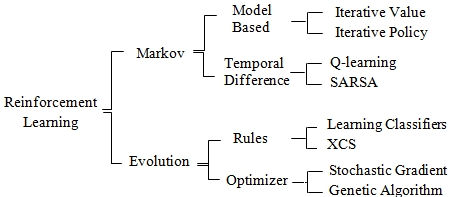Taxonomy of reinforcement learning algorithms

This is a brief overview of machine learning algorithms with a suggested, approximate taxonomy. There are almost as many ways to introduce machine learning as there are data and computer scientists. We encourage you to browse through the list of references at the end of the book to find the documentation appropriate to your level of interest and understanding.

## Don't reinvent the wheel!

There are numerous robust, accurate, and efficient Java libraries for mathematics, linear algebra, or optimization that have been widely used for many years:

There is absolutely no need to rewrite, debug, and test these components in Scala. Developers should consider creating a wrapper or interface to his/her favorite and reliable Java library. The book leverages the Apache Commons Math library for some specific linear algebra algorithms.

## Tools and frameworks

Before getting your hands dirty, you need to download and deploy a minimum set of tools and libraries; there is no need to reinvent the wheel after all. A few key components have to be installed in order to compile and run the source code described throughout the book. We focus on open source and commonly available libraries, although you are invited to experiment with equivalent tools of your choice. The learning curve for the frameworks described here is minimal.

### Java

The code described in this book has been tested with JDK 1.7.0_45 and JDK 1.8.0_25 on Windows x64 and Mac OS X x64. You need to install the Java Development Kit if you have not already done so. Finally, the `JAVA_HOME`, `PATH`, and `CLASSPATH` environment variables have to be updated accordingly.

### Scala

The code has been tested with Scala 2.10.4 and 2.11.4. We recommend that you use Scala Version 2.10.4 or higher with SBT 0.13 or higher. Let's assume that Scala runtime (REPL) and libraries have been properly installed and the `SCALA_HOME` and `PATH` environment variables have been updated.

The description and installation instructions of the Scala plugin for Eclipse (version 4.0 or higher) are available at http://scala-ide.org/docs/user/gettingstarted.html. You can also download the Scala plugin for IntelliJ IDEA (version 13 or higher) from the JetBrains website at http://confluence.jetbrains.com/display/SCA/.

The ubiquitous Simple Build Tool (SBT) will be our primary building engine. The syntax of the build file, `sbt/build.sbt`, conforms to the Version 0.13 and is used to compile and assemble the source code presented throughout the book. Sbt can be downloaded as part of Typesafe activator or directly from http://www.scala-sbt.org/download.html.

### Apache Commons Math

Apache Commons Math is a Java library used for numerical processing, algebra, statistics, and optimization [1:6].

#### Description

This is a lightweight library that provides developers with a foundation of small, ready-to-use Java classes that can be easily weaved into a machine learning problem. The examples used throughout the book require Version 3.5 or higher.

The math library supports the following:

• Functions, differentiation, and integral and ordinary differential equations

• Statistics distributions

• Linear and nonlinear optimization

• Dense and sparse vectors and matrices

• Curve fitting, correlation, and regression

#### Installation

The installation and deployment of the Apache Commons Math library are quite simple. The steps are as follows:

2. Download the latest `.jar` files to the binary section, `commons-math3-3.5-bin.zip` (for instance, for Version 3.5).

3. Unzip and install the `.jar` file.

4. Add `commons-math3-3.5.jar` to the classpath as follows:

• For Mac OS X: `export CLASSPATH=\$CLASSPATH:/Commons_Math_path/commons-math3-3.5.jar`

• For Windows: Go to system Properties | Advanced system settings | Advanced | Environment Variables, then edit the `CLASSPATH` variable

5. Add the `commons-math3-3.5.jar` file to your IDE environment if needed (that is, for Eclipse, go to Project | Properties | Java Build Path | Libraries | Add External JARs and for IntelliJ IDEA, go to File | Project Structure | Project Settings | Libraries).

You can also download `commons-math3-3.5-src.zip` from the Source section.

### JFreeChart

JFreeChart is an open source chart and plotting Java library, widely used in the Java programmer community. It was originally created by David Gilbert [1:7].

#### Description

The library supports a variety of configurable plots and charts (scatter, dial, pie, area, bar, box and whisker, stacked, and 3D). We use JFreeChart to display the output of data processing and algorithms throughout the book, but you are encouraged to explore this great library on your own, as time permits.

#### Licensing

It is distributed under the terms of the GNU Lesser General Public License (LGPL), which permits its use in proprietary applications.

#### Installation

To install and deploy JFreeChart, perform the following steps:

2. Unzip and deploy the `.jar` file.

3. Add `jfreechart-1.0.17.jar` (for Version 1.0.17) to the classpath as follows:

• For Mac OS X: `export CLASSPATH=\$CLASSPATH:/JFreeChart_path/jfreechart-1.0.17.jar`

• For Windows: Go to system Properties | Advanced system settings | Advanced | Environment Variables, then edit the `CLASSPATH` variable

4. Add the `jfreechart-1.0.17.jar` file to your IDE environment, if needed

### Other libraries and frameworks

Libraries and tools that are specific to a single chapter are introduced along with the topic. Scalable frameworks are presented in the last chapter along with the instructions to download them. Libraries related to the conditional random fields and support vector machines are described in their respective chapters.

### Note

Why not use the Scala algebra and numerical libraries?

Libraries such as Breeze, ScalaNLP, and Algebird are interesting Scala frameworks for linear algebra, numerical analysis, and machine learning. They provide even the most seasoned Scala programmer with a high-quality layer of abstraction. However, this book is designed as a tutorial that allows developers to write algorithms from the ground up using existing or legacy Java libraries [1:8].

## Source code

The Scala programming language is used to implement and evaluate the machine learning techniques covered in Scala for Machine Learning. However, the source code snippets are reduced to the strict minimum essential to the understanding of machine learning algorithms discussed throughout the book. The formal implementation of these algorithms is available on the website of Packt Publishing (http://www.packtpub.com).

### Tip

You can download the example code files for all Packt books you have purchased from your account at http://www.packtpub.com. If you purchased this book elsewhere, you can visit http://www.packtpub.com/support and register to have the files e-mailed directly to you.

### Context versus view bounds

Most Scala classes discussed in the book are parameterized with the type associated with the discrete/categorical value (`Int`) or continuous value (`Double`). Context bounds would require that any type used by the client code has `Int` or `Double` as upper bounds:

```class A[T <: Int](param: Param)
class B[T <: Double](param: Param)```

Such a design introduces constraints on the client to inherit from simple types and to deal with covariance and contravariance for container types [1:9].

For this book, view bounds are used instead of context bounds because they only require an implicit conversion to the parameterized type to be defined:

```class A[T <: AnyVal](param: Param)(implicit f: T => Int)
class C[T < : AnyVal](param: Param)(implicit f: T => Float)```

### Note

View bound deprecation

The notation for the view bound, `T <% Double`, is being deprecated in Scala 2.11 and higher. The `class A[T <% Float]` declaration is the short notation for `class A[T](implicit f: T => Float)`.

### Presentation

For the sake of readability of the implementation of algorithms, all nonessential code such as error checking, comments, exceptions, or imports are omitted. The following code elements are omitted in the code snippet presented in the book:

• Code documentation:

```// …..
/* … */```
• Validation of class parameters and method arguments:

`require( Math.abs(x) < EPS, " …")`
• Class qualifiers and scope declaration:

```final protected class SVM { … }
private[this] val lsError = …```
• Method qualifiers:

`final protected def dot: = …`
• Exceptions:

```try {
correlate …
} catch {
case e: MathException => ….
}
Try {    .. } match {
case Success(res) =>
case Failure(e => ..
}```
• Logging and debugging code:

```private val logger = Logger.getLogger("..")
logger.info( … )```
• Nonessential annotation:

```@inline def main = ….
@throw(classOf[IllegalStateException])```
• Nonessential methods

The complete list of Scala code elements omitted in the code snippets in this book can be found in the Code snippets format section in the Appendix A, Basic Concepts.

### Primitives and implicits

The algorithms presented in this book share the same primitive types, generic operators, and implicit conversions.

#### Primitive types

For the sake of readability of the code, the following primitive types will be used:

```type DblPair = (Double, Double)
type DblArray = Array[Double]
type DblMatrix = Array[DblArray]
type DblVector = Vector[Double]
type XSeries[T] = Vector[T]         // One dimensional vector
type XVSeries[T] = Vector[Array[T]] // multi-dimensional vector```

The times series introduced in the Time series in Scala section in Chapter 3, Data Preprocessing, is implemented as `XSeries[T]` or `XVSeries[T]` of a parameterized `T` type.

### Note

Make a note of these six types; they are used throughout the book.

#### Type conversions

Implicit conversion is an important feature of the Scala programming language. It allows developers to specify a type conversion for an entire library in a single place. Here are a few of the implicit type conversions that are used throughout the book:

```object Types {
Object ScalaMl {
implicit def double2Array(x: Double): DblArray =
Array[Double](x)
implicit def dblPair2Vector(x: DblPair): Vector[DblPair] =
Vector[DblPair](x._1,x._2)
...
}
}```

### Note

Library-specific conversion

The conversion between the primitive type listed here and types introduced in a particular library (such as, the Apache Commons Math library) are described in the relevant chapters.

### Immutability

It is usually a good idea to reduce the number of states of an object. A method invocation transitions an object from one state to another. The larger the number of methods or states, the more cumbersome the testing process becomes.

There is no point in creating a model that is not defined (trained). Therefore, making the training of a model as part of the constructor of the class it implements makes a lot of sense. Therefore, the only public methods of a machine learning algorithm are as follows:

• Classification or prediction

• Validation

• Retrieval of model parameters (weights, latent variables, hidden states, and so on), if needed

### Performance of Scala iterators

The evaluation of the performance of Scala high-order iterative methods is beyond the scope of this book. However, it is important to be aware of the trade-off of each method.

The `for` construct is to be avoided as a counting iterator. It is designed to implement the for-comprehensive monad (`map` and `flatMap`). The source code presented in this book uses the high-order `foreach` method instead.

## Let's kick the tires

This final section introduces the key elements of the training and classification workflow. A test case using a simple logistic regression is used to illustrate each step of the computational workflow.

### An overview of computational workflows

In its simplest form, a computational workflow to perform runtime processing of a dataset is composed of the following stages:

2. Splitting the dataset for parallel data processing.

3. Preprocessing data using filtering techniques, analysis of variance, and applying penalty and normalization functions whenever necessary.

4. Applying the model—either a set of clusters or classes—to classify new data.

5. Assessing the quality of the model.

A similar sequence of tasks is used to extract a model from a training dataset:

2. Splitting the dataset for parallel data processing.

3. Applying filtering techniques, analysis of variance, and penalty and normalization functions to the raw dataset whenever necessary.

4. Selecting the training, testing, and validation set from the cleansed input data.

5. Extracting key features and establishing affinity between a similar group of observations using clustering techniques or supervised learning algorithms.

6. Reducing the number of features to a manageable set of attributes to avoid overfitting the training set.

7. Validating the model and tuning the model by iterating steps 5, 6, and 7 until the error meets a predefined convergence criteria.

8. Storing the model in a file or database so that it can be applied to future observations.

Data clustering and data classification can be performed independent of each other or as part of a workflow that uses clustering techniques at the preprocessing stage of the training phase of a supervised learning algorithm. Data clustering does not require a model to be extracted from a training set, while classification can be performed only if a model has been built from the training set. The following image gives an overview of training, classification, and validation: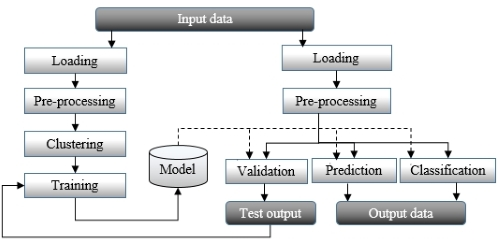A generic data flow for training and running a model

The preceding diagram is an overview of a typical data mining processing pipeline. The first phase consists of extracting the model through clustering or training of a supervised learning algorithm. The model is then validated against test data for which the source is the same as the training set but with different observations. Once the model is created and validated, it can be used to classify real-time data or predict future behavior. Real-world workflows are more complex and require dynamic configuration to allow experimentation of different models. Several alternative classifiers can be used to perform a regression and different filtering algorithms are applied against input data, depending on the latent noise in the raw data.

### Writing a simple workflow

This book relies on financial data to experiment with different learning strategies. The objective of the exercise is to build a model that can discriminate between volatile and nonvolatile trading sessions for stock or commodities. For the first example, we select a simplified version of the binomial logistic regression as our classifier as we treat stock-price-volume action as a continuous or pseudo-continuous process.

### Note

An introduction to the logistic regression

Logistic regression is explained in depth in the Logistic regression section in Chapter 6, Regression and Regularization. The model treated in this example is the simple binomial logistic regression classifier for two-dimension observations.

The steps for classification of trading sessions according to their volatility and volume is as follows:

1. Scoping the problem

3. Preprocessing raw data

4. Discovering patterns, whenever possible

5. Implementing the classifier

6. Evaluating the model

#### Step 1 – scoping the problem

The objective is to create a model for stock price using its daily trading volume and volatility. Throughout the book, we will rely on financial data to evaluate and discuss the merits of different data processing and machine learning methods. In this example, the data is extracted from Yahoo Finances using the CSV format with the following fields:

• Date

• Price at open

• Highest price in the session

• Lowest price in the session

• Price at session close

• Volume

• Adjust price at session close

The `YahooFinancials` enumerator extracts the historical daily trading information from the Yahoo finance site:

```type Fields = Array[String]
object YahooFinancials extends Enumeration {
type YahooFinancials = Value
val DATE, OPEN, HIGH, LOW, CLOSE, VOLUME, ADJ_CLOSE = Value

def toDouble(v: Value): Fields => Double =   //1
(s: Fields) => s(v.id).toDouble
def toDblArray(vs: Array[Value]): Fields => DblArray = //2
(s: Fields) => vs.map(v => s(v.id).toDouble)
…
}```

The `toDouble` method converts an array of string into a single value (line `1`) and `toDblArray` converts an array of string into an array of values (line `2`). The `YahooFinancials` enumerator is described in the Data sources section in Appendix A, Basic Concepts in detail.

Let's create a simple program that loads the content of the file, executes some simple preprocessing functions, and creates a simple model. We selected the CSCO stock price between January 1, 2012 and December 1, 2013 as our data input.

Let's consider the two variables, price and volume, as shown in the following screenshot. The top graph displays the variation of the price of Cisco stock over time and the bottom bar chart represents the daily trading volume on Cisco stock over time: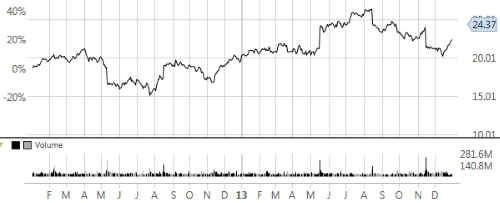Price-Volume action for Cisco stock 2012-2013

The second step is loading the dataset from a local or remote data storage. Typically, large datasets are loaded from a database or distributed filesystems such as Hadoop Distributed File System (HDFS). The `load` method takes an absolute pathname, `extract`, and transforms the input data from a file into a time series of a `Vector[DblPair]` type:

```def load(fileName: String): Try[Vector[DblPair]] = Try {
val src =  Source.fromFile(fileName)  //3
val data = extract(src.getLines.map(_.split(",")).drop(1)) //4
src.close //5
data
}```

The data file is extracted through an invocation of the `Source.fromFile` static method (line `3`), and then the fields are extracted through a map before the header (first row in the file) is removed using `drop` (line `4`). The file has to be closed to avoid leaking of the file handle (line `5`).

### Note

Data extraction

The `Source.fromFile.getLines.map` invocation pipeline method returns an iterator that can be traversed only once.

The purpose of the `extract` method is to generate a time series of two variables (relative stock volatility and relative stock daily trading volume):

```def extract(cols: Iterator[Array[String]]): XVSeries[Double]= {
val features = Array[YahooFinancials](LOW, HIGH, VOLUME) //6
val conversion = YahooFinancials.toDblArray(features)  //7
cols.map(c => conversion(c)).toVector
.map(x => Array[Double](1.0 - x(0)/x(1), x(2)))  //8
}```

The only purpose of the `extract` method is to convert the raw textual data into a two-dimensional time series. The first step consists of selecting the three features to extract `LOW` (the lowest stock price in the session), `HIGH` (the highest price in the session), and `VOLUME` (trading volume for the session) (line `6`). This feature set is used to convert each line of fields into a corresponding set of three values (line `7`). Finally, the feature set is reduced to the following two variables (line `8`):

• Relative volatility of the stock price in a session: 1.0 – LOW/HIGH

• Trading volume for the stock in the session: VOLUME

### Note

A long pipeline of Scala high-order methods make the code and underlying code quite difficult to read. It is recommended that you break down long chains of method calls, such as the following:

`val cols = Source.fromFile.getLines.map(_.split(",")).toArray.drop(1)`

We can break down method calls into several steps as follows:

```val lines = Source.fromFile.getLines
val fields = lines.map(_.split(",")).toArray
val cols = fields.drop(1)```

We strongly encourage you to consult the excellent guide Effective Scala, written by Marius Eriksen from Twitter. This is definitively a must read for any Scala developer [1:10].

#### Step 3 – preprocessing the data

The next step is to normalize the data in the range [0.0, 1.0] to be trained by the binomial logistic regression. It is time to introduce an immutable and flexible normalization class.

##### Immutable normalization

The logistic regression relies on the sigmoid curve or logistic function is described in the Logistic function section in Chapter 6, Regression and Regularization. The logistic functions are used to segregate training data into classes. The output value of the logistic function ranges from 0 for x = - INFINITY to 1 for x = + INFINITY. Therefore, it makes sense to normalize the input data or observation over [0, 1].

### Note

Normalize or not normalize?

The purpose of normalizing data is to impose a single range of values for all the features, so the model does not favor any particular feature. Normalization techniques include linear normalization and Z-score. Normalization is an expensive operation that is not always needed.

The normalization is a linear transformation of the raw data that can be generalized to any range [l, h].

### Note

Linear normalization

M2: [0, 1] Normalization of features {xi} with minimum xmin and maximum xmax values: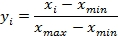M3: [l, h] Normalization of features {xi}: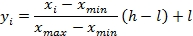The normalization of input data in supervised learning has a specific requirement: the classification and prediction of new observations have to use the normalization parameters (min and max) extracted from the training set, so all the observations share the same scaling factor.

Let's define the `MinMax` normalization class. The class is immutable: the minimum, `min`, and maximum, `max`, values are computed within the constructor. The class takes a time series of a parameterized `T` type and values as arguments (line `8`). The steps of the normalization process are defined as follows:

1. Initialize the minimum values for a given time series during instantiation (line `9`).

2. Compute the normalization parameters (line `10`) and normalize the input data (line `11`).

3. Normalize any new data points reusing the normalization parameters (line `14`):

```class MinMax[T <: AnyVal](val values: XSeries[T]) (f : T => Double) { //8
val zero = (Double.MaxValue, -Double.MaxValue)
val minMax = values./:(zero)((mM, x) => { //9
val min = mM._1
val max = mM._2
(if(x < min) x else min, if(x > max) x else max)
})
case class ScaleFactors(low:Double ,high:Double, ratio: Double)
var scaleFactors: Option[ScaleFactors] = None //10

def min = minMax._1
def max = minMax._2
def normalize(low: Double, high: Double): DblVector //11
def normalize(value: Double): Double
}```

The class constructor computes the tuple of minimum and maximum values, `minMax`, using a fold (line `9`). The `scaleFactors` scaling parameters are computed during the normalization of the time series (line `11`), which are described as follows. The `normalize` method initializes the scaling factor parameters (line `12`) before normalizing the input data (line `13`):

```def normalize(low: Double, high: Double): DblVector =
setScaleFactors(low, high).map( scale => { //12
values.map(x =>(x - min)*scale.ratio + scale.low) //13
}).getOrElse(/* … */)

def setScaleFactors(l: Double, h: Double): Option[ScaleFactors]={
// .. error handling code
Some(ScaleFactors(l, h, (h - l)/(max - min))
}```

Subsequent observations use the same scaling factors extracted from the input time series in `normalize` (line `14`):

```def normalize(value: Double):Double = setScaleFactors.map(scale =>
if(value < min) scale.low
else if (value > max) scale.high
else (value - min)* scale.high + scale.low
).getOrElse( /* … */)```

The `MinMax` class normalizes single variable observations.

### Note

The statistics class

The class that extracts the basic statistics from a `Stats` dataset, which is introduced in the Profiling data section in Chapter 2, Hello World!, inherits the `MinMax` class.

The test case with the binomial logistic regression uses a multiple variable normalization, implemented by the `MinMaxVector` class, which takes observations of the `XVSeries[Double]` type as inputs:

```class MinMaxVector(series: XVSeries[Double]) {
val minMaxVector: Vector[MinMax[Double]] = //15
series.transpose.map(new MinMax[Double](_))
def normalize(low: Double, high: Double): XVSeries[Double]
}```

The constructor of the `MinMaxVector` class transposes the vector of array of observations in order to compute the minimum and maximum value for each dimension (line `15`).

#### Step 4 – discovering patterns

The price action chart has a very interesting characteristic.

##### Analyzing data

At a closer look, a sudden change in price and increase in volume occurs about every three months or so. Experienced investors will undoubtedly recognize that these price-volume patterns are related to the release of quarterly earnings of Cisco. Such a regular but unpredictable pattern can be a source of concern or opportunity if risk can be properly managed. The strong reaction of the stock price to the release of corporate earnings may scare some long-term investors while enticing day traders.

The following graph visualizes the potential correlation between sudden price change (volatility) and heavy trading volume: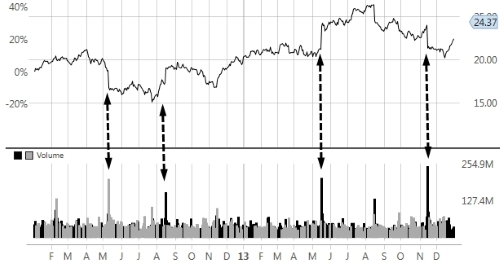Price-volume correlation for the Cisco stock 2012-2013

The next section is not required for the understanding of the test case. It illustrates the capabilities of JFreeChart as a simple visualization and plotting library.

##### Plotting data

Although charting is not the primary goal of this book, we thought that you will benefit from a brief introduction to JFreeChart.

### Note

Plotting classes

This section illustrates a simple Scala interface to JFreeChart Java classes. Reading this is not required for the understanding of machine learning. The visualization of the results of a computation is beyond the scope of this book.

Some of the classes used in visualization are described in the Appendix A, Basic Concepts.

The dataset (volatility and volume) is converted into internal JFreeChart data structures. The `ScatterPlot` class implements a simple configurable scatter plot with the following arguments:

• `config`: This includes information, labels, fonts, and so on, of the plot

• `theme`: This is the predefined theme for the plot (black, white background, and so on)

The code will be as follows:

```class ScatterPlot(config: PlotInfo, theme: PlotTheme) { //16
def display(xy: Vector[DblPair], width: Int, height) //17
def display(xt: XVSeries[Double], width: Int, height)
// ….
}```

The `PlotTheme` class defines a specific theme or preconfiguration of the chart (line 16). The class offers a set of `display` methods to accommodate a wide range of data structures and configuration (line `17`).

### Note

Visualization

The JFreeChart library is introduced as a robust charting tool. The code related to plots and charts is omitted from the book in order to keep the code snippets concise and dedicated to machine learning. On a few occasions, output data is formatted as a CSV file to be imported into a spreadsheet.

The `ScatterPlot.display` method is used to display the normalized input data used in the binomial logistic regression as follows:

```val plot = new ScatterPlot(("CSCO 2012-2013",
"Session High - Low", "Session Volume"), new BlackPlotTheme)
plot.display(volatility_vol, 250, 340)```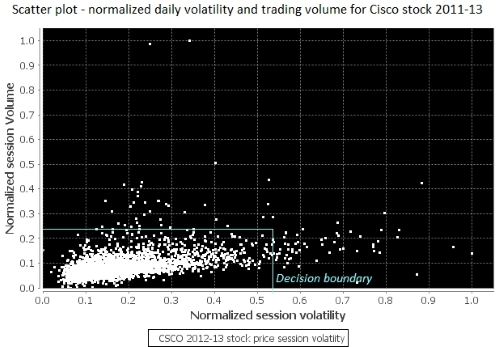A scatter plot of volatility and volume for the Cisco stock 2012-2013

The scatter plot shows a level of correlation between session volume and session volatility and confirms the initial finding in the stock price and volume chart. We can leverage this information to classify trading sessions by their volatility and volume. The next step is to create a two class model by loading a training set, observations, and expected values, into our logistic regression algorithm. The classes are delimited by a decision boundary (also known as a hyperplane) drawn on the scatter plot.

Visualizing labels—the normalized variation of the stock price between the opening and closing of the trading session is selected as the label for this classifier.

#### Step 5 – implementing the classifier

The objective of this training is to build a model that can discriminate between volatile and nonvolatile trading sessions. For the sake of the exercise, session volatility is defined as the relative difference between the session highest price and lower price. The total trading volume within a session constitutes the second parameter of the model. The relative price movement within a trading session (that is, closing price/open price - 1) is our expected values or labels.

Logistic regression is commonly used in statistics inference.

### Tip

M4: Logistic regression model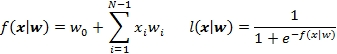The first weight w0 is known as the intercept. The binomial logistic regression is described in the Logistic regression section in Chapter 6, Regression and Regularization, in detail.

The following implementation of the binomial logistic regression classifier exposes a single `classify` method to comply with our desire to reduce the complexity and life cycle of objects. The model `weights` parameters are computed during training when the `LogBinRegression` class/model is instantiated. As mentioned earlier, the sections of the code nonessential to the understanding of the algorithm are omitted.

The `LogBinRegression` constructor has five arguments (line `18`):

• `obsSet`: These are vector observations that represent volume and volatility

• `expected`: This is a vector of expected values

• `maxIters`: This is the maximum number of iterations allowed for the optimizer to extract the regression weights during training

• `eta`: This is the learning or training rate

• `eps`: This is the maximum value of the error (predicted—expected) for which the model is valid

The code is as follows:

```class LogBinRegression(
obsSet: Vector[DblArray],
expected: Vector[Int],
maxIters: Int,
eta: Double,
eps: Double) {  //18

val model: LogBinRegressionModel = train  //19
def classify(obs: DblArray): Try[(Int, Double)]   //20
def train: LogBinRegressionModel
def intercept(weights: DblArray): Double
…
}```

The `LogBinRegressionModel` model is generated through training during the instantiation of the `LogBinRegression` logistic regression class (line `19`):

`case class LogBinRegressionModel(val weights: DblArray)`

The model is fully defined by its weights, as described in the mathematical formula M3. The `weights(0)` intercept represents the mean value of the prediction for observations for which variables are zero. The intercept does not have any specific meaning for most of the cases and it is not always computable.

### Note

Intercept or not intercept?

The intercept corresponds to the value of weights when the observations have null values. It is a common practice to estimate, whenever possible, the intercept for binomial linear or logistic regression independently from the slope of the model in the minimization of the error function. The multinomial regression models treat the intercept or weight w0 as part of the regression model, as described in the Ordinary least squares regression section of Chapter 6, Regression and Regularization.

The code will be as follows:

```def intercept(weights: DblArray): Double = {
val zeroObs = obsSet.filter(!_.exists( _ > 0.01))
if( zeroObs.size > 0)
zeroObs.aggregate(0.0)((s,z) => s + dot(z, weights),
_ + _ )/zeroObs.size
else 0.0
}```

The `classify` methods takes new observations as inputs and compute the index of the classes (0 or 1) the observations belong to and the actual likelihood (line `20`).

##### Selecting an optimizer

The goal of the training of a model using expected values is to compute the optimal weights that minimizes the error or cost function. We select the batch gradient descent algorithm to minimize the cumulative error between the predicted and expected values for all the observations. Although there are quite a few alternative optimizers, the gradient descent is quite robust and simple enough for this first chapter. The algorithm consists of updating the weights wi of the regression model by minimizing the cost.

### Note

Cost function

M5: Cost (or compound error = predicted – expected):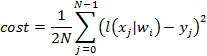M6: The batch gradient descent method to update model weights wi is as follows: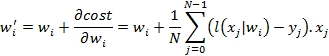For those interested in learning about of optimization techniques, the Summary of optimization techniques section in the Appendix A, Basic Concepts presents an overview of the most commonly used optimizers. The batch descent gradient method is also used for the training of the multilayer perceptron (refer to The training epoch section under The multilayer perceptron section in Chapter 9, Artificial Neural Networks).

The execution of the batch gradient descent algorithm follows these steps:

1. Initialize the weights of the regression model.

2. Shuffle the order of observations and expected values.

3. Aggregate the cost or error for the entire observation set.

4. Update the model weights using the cost as the objective function.

5. Repeat from step 2 until either the maximum number of iterations is reached or the incremental update of the cost is close to zero.

The purpose of shuffling the order of the observations between iterations is to avoid the minimization of the cost reaching a local minimum.

### Tip

The stochastic gradient descent is a variant of the gradient descent that updates the model weights after computing the error on each observation. Although the stochastic gradient descent requires a higher computation effort to process each observation, it converges toward the optimal value of weights fairly quickly after a small number of iterations. However, the stochastic gradient descent is sensitive to the initial value of the weights and the selection of the learning rate, which is usually defined by an adaptive formula.

##### Training the model

The `train` method consists of iterating through the computation of the weight using a simple descent gradient method. The method computes `weights` and returns an instance of the `LogBinRegressionModel` model:

```def train: LogBinRegressionModel = {
val nWeights = obsSet.head.length + 1  //21
val init = Array.fill(nWeights)(Random.nextDouble )  //22
new LogBinRegressionModel(weights)   //23
}```

The `train` method extracts the number of weights, `nWeights`, for the regression model as the number of variables in each observation + 1 (line `21`). The method initializes `weights` with random values over [0, 1] (line `22`). The weights are computed through the tail recursive `gradientDescent` method, and the method returns a new model for the binomial logistic regression (line `23`).

### Tip

Unwrapping values from Try

It is usually not recommended to invoke the `get` method to a `Try` value, unless it is enclosed in a `Try` statement. The best course of action is to do the following:

1. Catch the failure with `match{ case Success(m) => ..case Failure(e) =>}`

2. Extract the `getOrElse( /* … */ )` result safely

3. Propagate the results as a `Try` type `map( _.m)`

Let's take a look at the computation for `weights` through the minimization of the cost function in the `gradientDescent` method:

```type LabelObs = Vector[(DblArray, Int)]

@tailrec
obsAndLbl: LabelObs,
cost: Double,
nIters: Int,
weights: DblArray): DblArray = {  //24

if(nIters >= maxIters)
throw new IllegalStateException("..")//25
val shuffled = shuffle(obsAndLbl)   //26
val errorGrad = shuffled.map{ case(x, y) => {  //27
val error = sigmoid(dot(x, weights)) - y
(error, x.map( _ * error))  //28
}}.unzip

val scale = 0.5/obsAndLbl.size
.aggregate(0.0)((s,c) =>s + c*c, _ + _ )*scale
val relativeError = cost/newCost - 1.0

if( Math.abs(relativeError) < eps)  weights  //30
else {
val derivatives = Vector[Double](1.0) ++
val newWeights = weights.zip(derivatives)
.map{ case (w, df) => w - eta*df)  //32
newWeights.copyToArray(weights)
}
}```

The `gradientDescent` method recurses on the vector of pairs (observations and expected values), `obsAndLbl`, `cost`, and the model `weights` (line `24`). It throws an exception if the maximum number of iterations allowed for the optimization is reached (line `25`). It shuffles the order of the observations (line `26`) before computing the `errorGrad` derivatives of the cost over each weights (line `27`). The computation of the derivative of the cost (or error = predicted value – expected value) in formula M5 returns a pair of cumulative cost and derivative values using the formula (line `28`).

Next, the method computes the overall compound cost using the formula M4 (line `29`), converts it to a relative incremental `relativeError` cost that is compared to the `eps` convergence criteria (line `30`). The method extracts `derivatives` of cost over weights by transposing the matrix of errors, and then prepends the bias `1.0` value to match the array of weights (line `31`).

### Note

Bias value

The purpose of the bias value is to prepend `1.0` to the vector of observation so it can be directly processed (for example, zip and dot) with the weights. For instance, a regression model for two-dimensional observations (x, y) has three weights (w0, w1, w2). The bias value +1 is prepended to the observations to compute the predicted value 1.0: w0 + x.w1, + y.w2.

This technique is used in the computation of the activation function of the multilayer perceptron, as described in the The multilayer perceptron section in Chapter 9, Artificial Neural Networks.

The formula M6 updates the weights for the next iteration (line `32`) before invoking the method with new weights, cost, and iteration count (line `33`).

Let's take a look at the shuffling of the order of observations using a random sequence generator. The following implementation is an alternative to the Scala standard library method `scala.util.Random.shuffle` for shuffling elements of collections. The purpose is to change the order of observations and labels between iterations in order to prevent the optimizer to reach a local minimum. The `shuffle` method permutes the order in the `labelObs` vector of observations by partitioning it into segments of random size and reversing the order of the other segment:

```val SPAN = 5
def shuffle(labelObs: LabelObs): LabelObs = {
shuffle(new ArrayBuffer[Int],0,0).map(labelObs( _ )) //34
}```

Once the order of the observations is updated, the vector of pair (observations, labels) is easily built through a map (line `34`). The actual shuffling of the index is performed in the following `shuffle` recursive function:

```val maxChunkSize = Random.nextInt(SPAN)+2  //35

@tailrec
def shuffle(indices: ArrayBuffer[Int], count: Int, start: Int):
Array[Int] = {
val end = start + Random.nextInt(maxChunkSize) //36
val isOdd = ((count & 0x01) != 0x01)
if(end >= sz)
indices.toArray ++ slice(isOdd, start, sz) //37
else
shuffle(indices ++ slice(isOdd, start, end), count+1, end)
}```

The maximum size of partition of the `maxChunkSize` vector observations is randomly computed (line `35`). The method extracts the next slice (`start`, `end`) (line `36`). The slice is either added to the existing indices vector and returned once all the observations have been shuffled (line `37`) or passed to the next invocation.

The `slice` method returns an array of indices over the range (`start`, `end`) either in the right order if the number of segments processed is odd, or in reverse order if the number of segment processed is even:

```def slice(isOdd: Boolean, start: Int, end: Int): Array[Int] = {
val r = Range(start, end).toArray
(if(isOdd) r else r.reverse)
}```

### Note

Iterative versus tail recursive computation

The tail recursion in Scala is a very efficient alternative to the iterative algorithm. Tail recursion avoids the need to create a new stack frame for each invocation of the method. It is applied to the implementation of many machine learning algorithms presented throughout the book.

In order to train the model, we need to label the input data. The labeling process consists of associating the relative price movement during a session (price at close/price at open – 1) with one of the following two configurations:

The two classes of training observations are segregated by a decision boundary drawn on the scatter plot in the previous section. The labeling process is usually quite cumbersome and should be automated as much as possible.

### Note

Automated labeling

Although quite convenient, automated creation of training labels is not without risk as it may mislabel singular observations. This technique is used in this test for convenience, but it is not recommended unless a domain expert reviews the labels manually.

##### Classifying observations

Once the model is successfully created through training, it is available to classify new observation. The runtime classification of observations using the binomial logistic regression is implemented by the `classify` method:

```def classify(obs: DblArray): Try[(Int, Double)] =
val linear = dot(obs, model.weights)  //37
val prediction = sigmoid(linear)
(if(linear > 0.0) 1 else 0, prediction) //38
})```

The method applies the logistic function to the linear inner product, `linear`, of the new `obs` and `weights` observations of the model (line `37`). The method returns the tuple (the predicted class of the observation {0, 1}, prediction value) where the class is defined by comparing the prediction to the boundary value `0.0` (line `38`).

The computation of the `dot` product of weights and observations uses the bias value as follows:

```def dot(obs: DblArray, weights: DblArray): Double =
weights.zip(Array[Double](1.0) ++ obs)
.aggregate(0.0){case (s, (w,x)) => s + w*x, _ + _ }```

The alternative implementation of the `dot` product of weights and observations consists of extracting the first `w.head` weight:

```def `dot`(x: DblArray, w: DblArray): Double =
x.zip(w.drop(1)).map {case (_x,_w) => _x*_w}.sum + w.head```

The `dot` method is used in the `classify` method.

#### Step 6 – evaluating the model

The first step is to define the configuration parameters for the test: the maximum number of `NITERS` iterations, the `EPS` convergence criteria, the `ETA` learning rate, the decision boundary used to label the `BOUNDARY` training observations, and the path to the training and test sets:

```val NITERS = 800; val EPS = 0.02; val ETA = 0.0001
val path_training = "resources/data/chap1/CSCO.csv"
val path_test = "resources/data/chap1/CSCO2.csv"```

The various activities of creating and testing the model, loading, normalizing data, training the model, loading, and classifying test data is organized as a workflow using the monadic composition of the `Try` class:

```for {
minMaxVec <- Try(new MinMaxVector(volatilityVol))    //40
normVolatilityVol <- Try(minMaxVec.normalize(0.0,1.0))//41
classifier <- logRegr(normVolatilityVol)    //42
normTestValue0 <- minMaxVec.normalize(testValues(0))  //44
class0 <- classifier.classify(normTestValue0)   //45
normTestValue1 <- minMaxVec.normalize(testValues(1))
class1 <- classifier.classify(normTestValues1)
} yield {
val modelStr = model.toString
…
}```

First, the daily trading volatility and volume for the `volatilityVol` stock price is loaded from file (line `39`). The workflow initializes the multi-dimensional `MinMaxVec` normalizer (line `40`) and uses it to normalize the training set (line `41`). The `logRegr` method instantiates the binomial `classifier` logistic regression (line `42`). The `testValues` test data is loaded from file (line `43`), normalized using `MinMaxVec` already applied to the training data (line `44`), and classified (line `45`).

The `load` method extracts `data` (observations) of a `XVSeries[Double]` type from the file. The heavy lifting is done by the `extract` method (line `46`), and then the file handle is closed (line `47`) before returning the vector of raw observations:

```def load(fileName: String): Try[XVSeries[Double], XSeries[Double]] =  {
val src =  Source.fromFile(fileName)
val data = extract(src.getLines.map( _.split(",")).drop(1)) //46
src.close; data //47
}```

The private `logRegr` method has the following two purposes:

• Labeling automatically the `obs` observations to generate the `expected` values (line `48`)

• Initializing (instantiation and training of the model) the binomial logistic regression (line `49`)

The code is as follows:

```def logRegr(obs: XVSeries[Double]): Try[LogBinRegression] = Try {
val expected = normalize(labels._2).get  //48
new LogBinRegression(obs, expected, NITERS, ETA, EPS)  //49
}```

The method labels observations by evaluating if they belong to any one of the two classes delimited by the `BOUNDARY` condition, as illustrated in the scatter plot in a previous section.

### Note

Validation

The simple classification in this test case is provided for illustrating the runtime application of the model. It does not constitute a validation of the model by any stretch of imagination. The next chapter digs into validation methodologies (refer to the Assessing a model section in Chapter 2, Hello World!

The training run is performed with three different values of the learning rate. The following chart illustrates the convergence of the batch gradient descent in the minimization of the cost, given different values of learning rates: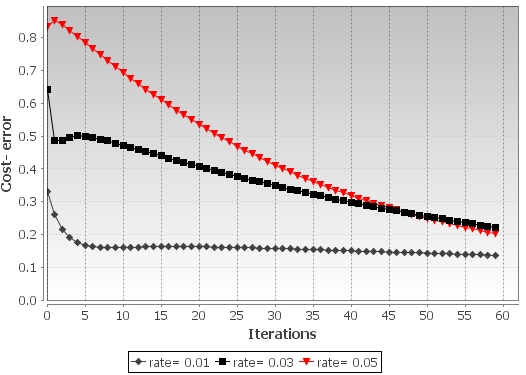Impact of the learning rate on the batch gradient descent on the convergence of the cost (error)

As expected, the execution of the optimizer with a higher learning rate produces a steepest descent in the cost function.

The execution of the test produces the following model:

iters = 495

weights: 0.859-3.6177923,-64.927832

input (0.0088, 4.10E7) normalized (0.063,0.061) class 1 prediction 0.515

input (0.0694, 3.68E8) normalized (0.517,0.641) class 0 prediction 0.001

### Note

The binomial logistic regression is merely used to illustrate the concept of training and prediction. It is described in the Logistic regression section in Chapter 6, Regression and Regularization in detail.

## Summary

I hope you enjoyed this introduction to machine learning. You learned how to leverage your skills in Scala programming to create a simple logistic regression program for predicting stock price/volume action. Here are the highlights of this introductory chapter:

• From monadic composition and high order collection methods for parallelization to configurability and reusability patterns, Scala is the perfect fit to implement data mining and machine learning algorithms for large-scale projects.

• There are many logical steps to create and deploy a machine learning model.

• The implementation of the binomial logistic regression classifier presented as part of the test case is simple enough to encourage you to learn how to write and apply more advanced machine learning algorithms.

To the delight of Scala programming aficionados, the next chapter will dig deeper into building a flexible workflow by leveraging monadic data transformation and stackable traits.